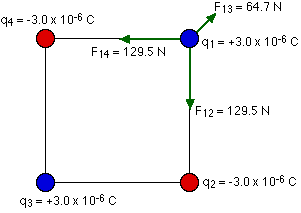## Friday, 3 October 2014

### Net force exerted on the charge the other three charges -ELECTRIC CHARGE PROBLEM

Four charges are arranged in a square with sides of length 2.5 cm. The two charges in the top right and bottom left corners are +3.0 x 10-6 C. The charges in the other two corners are -3.0 x 10-6 C. What is the net force exerted on the charge in the top right corner by the other three charges?To solve any problem like this, the simplest thing to do is to draw a good diagram showing the forces acting on the charge. You should also let your diagram handle your signs for you. Force is a vector, and any time you have a minus sign associated with a vector all it does is tell you about the direction of the vector. If you have the arrows giving you the direction on your diagram, you can just drop any signs that come out of the equation for Coulomb's law.
Consider the forces exerted on the charge in the top right by the other three:You have to be very careful to add these forces as vectors to get the net force. In this problem we can take advantage of the symmetry, and combine the forces from charges 2 and 4 into a force along the diagonal (opposite to the force from charge 3) of magnitude 183.1 N. When this is combined with the 64.7 N force in the opposite direction, the result is a net force of 118 N pointing along the diagonal of the square.The symmetry here makes things a little easier. If it wasn't so symmetric, all you'd have to do is split the vectors up in to x and y components, add them to find the x and y components of the net force, and then calculate the magnitude and direction of the net force from the components.